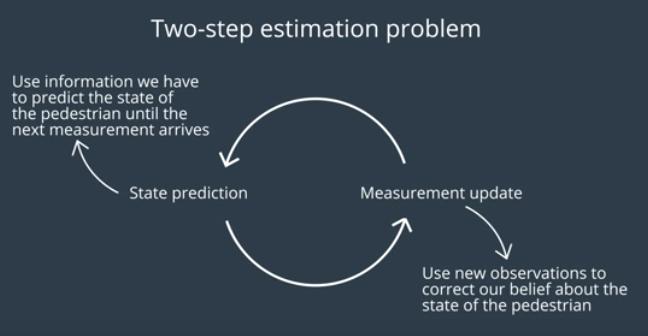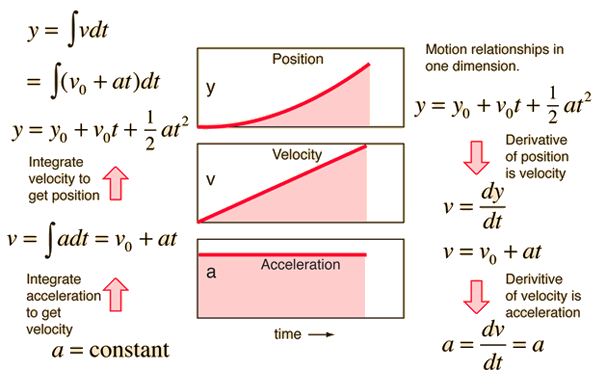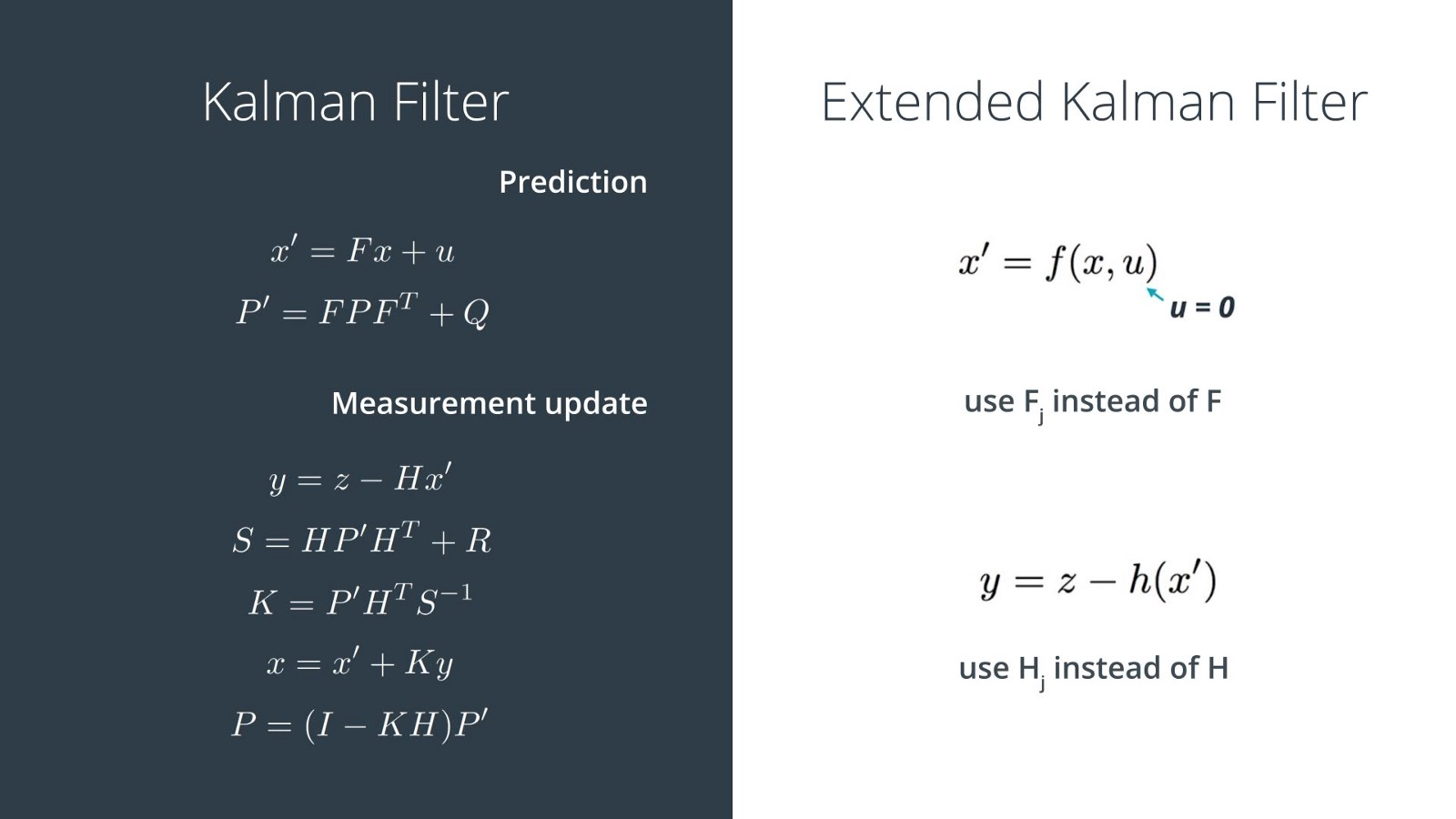You can find my final code and result here

# Problem

One of the most important part of a self driving car is know where it is located at any given time. However, it is hard to get an accurate position since the sensors has inherent error usually due to the technology (such as RADAR and LiDAR). For instance, GPS is rated for +/- 1m (3 ft) but 1 m error is enough for the car to hit things accidentally. This was systemic problem when NASA was developing rocket/missile controls. The error within the sensor varied too much where the missiles could not control itself correctly as intended.

# Solution

One solution to this problem is to use a Kalman filter. There are many great resources online that explain the theory and application of the Kalman filter so I will be mainly explaining my key takeaways that allowed me to understand it better. Kalman filter find it’s root from Bayesian statistic. One of the most important effect of the Bayes rule is that the more measurements you have for a given time, the lower the variance (more accurate) for your final measurement. Essentially, two (or more) noisy measurements will give you one (relatively) accurate measurement.

The Kalman filter involves two steps that runs perpetually:

1) Measurement Update 2) Motion Update## Measurement Update

Like the name implies, the measurement update is where you use the sensors to determine where you are. For instance, a self driving car can use GPS and wheel encoder to determine an approximate location. The raw measurement tends to be noisy but the variance can be greatly reduced using the Bayes rules using information that was obtained from the previous measurement (this will make more sense later).

## Motion Update

After the measurement update, you move the self driving car and predict the location of the self driving car. I use the word predict because the location is usually calculated rather then measured. Therefore, in order to perform this step, the state space has to be fully defined. This is just fancy way of saying that the physics of the system must be understood and defined. For instance, the position motion update is usually predicted using the velocity of the car that can be measured using an IMU or speedometer. Since we know the velocity, we can use our high school physics knowledge to predict the position.Source

This part is where the magic happens for the Kalman filter. By using the Bayes Rule, we can combine the measurements from the measurement step (the first posterior in the image) and the prediction from the motion step (the prior from the image) to get an accurate final result which will be the posterior used in the next motion update step. This shows you the powerful application of Bayesian statistics.

** PUT IMAGE HERE **

# Extended Kalman Filter

The extended Kalman filter (EKF) is the nonlinear version of a Kalman filter. I will not go into the math but the reason why EKF can accomdate for nonlinear functions is during the motion update phase, the state is linearized using an intermediate function (h(x’) shown in the image) that is usually defined using Taylor expansion. This makes the math a bit more complex but can be easily solved using the Jacobian matrix when programming it.# Unscented Kalman Filter

If you want a more thorough and good explanation, please read this medium post by Harveen Singh

I have been told that name for Unscented Kalman filter (UKF) stems from the idea that a UKF is a Kalman filter that doesn’t “stink” (or sucks and terrible for non-native English speakers). The UKF is similar to an EKF where it can account for nonlinear functions but takes it one step further which gives it better accuracy.

An EKF has induced error because when it maps it Gaussian distribution when it converts the non-linear to linear function, a lot of information can be lost. To alleviate this problem, UKF keeps track of data points in the distribution called sigma points. These points are important because you can gauge the effectiveness of the approximation. With this additional data, the final variance of the measurement is much more accurate since information from the previous step is retained better. Also, the sigma points alleviate the importance of the first measurement point which can cause an EKF to diverge.

The four additional steps for a UKF is the following:

1) Generate sigma points 2) Augment them during the nonlinear to linear process 3) Predict where the sigma points should be and compare them to how they are augmented 4) Update the measurement Gaussian distribution

# Putting it all together

For a self driving car application, the UKF is usually used for the senor fusion between RADAR and LiDAR data. The main reason is because RADAR gives relative velocity data where LiDAR gives positional data. By using the UKF, the measurement of the position of your car will be much more accurate.

## Measurement Update Step

``````void UKF::ProcessMeasurement(MeasurementPackage meas_package) {

/**

TODO:

Complete this function! Make sure you switch between lidar and radar

measurements.

*/

if (!is_initialized_) {

/**

TODO:

* Initialize the state ekf_.x_ with the first measurement.

* Create the covariance matrix.

* Remember: you'll need to convert radar from polar to cartesian coordinates.

*/

// first measurement

float px;

float py;

float vx;

float vy;

/**

Convert radar from polar to cartesian coordinates and initialize state.

*/

float rho = meas_package.raw_measurements_;

float phi = meas_package.raw_measurements_;

float rho_dot = meas_package.raw_measurements_;

px = rho * cos(phi);

py = rho * sin(phi);

vx = rho_dot * cos(phi);

vy = rho_dot * sin(phi);

x << px, py, vx, vy, 0;

}

else if (meas_package.sensor_type_ == MeasurementPackage::LASER) {

/**

Initialize state.

*/

px = meas_package.raw_measurements_;

py = meas_package.raw_measurements_;

x << px, py, 0, 0, 0;

}

previous_timestamp_ = meas_package.timestamp_;

// done initializing, no need to predict or update

is_initialized_ = true;

}

double dt = (meas_package.timestamp_ - previous_timestamp_) / 1000000.0; //dt - expressed in seconds

previous_timestamp_ = meas_package.timestamp_;

Prediction(dt);

/*****************************************************************************

* Update

****************************************************************************/

/**

TODO:

* Use the sensor type to perform the update step.

* Update the state and covariance matrices.

*/

} else {

UpdateLidar(meas_package);

}

}
``````

## Motion Update Step

``````void UKF::Prediction(double delta_t) {

/**

TODO:

Complete this function! Estimate the object's location. Modify the state

vector, x_. Predict sigma points, the state, and the state covariance matrix.

*/

MatrixXd Xsig_out;

GenerateSigmaPoints(&Xsig_out);

AugmentedSigmaPoints(&Xsig_out);

SigmaPointPrediction(delta_t,&Xsig_out);

PredictMeanAndCovariance(&Xsig_out);

}

void UKF::GenerateSigmaPoints(MatrixXd* Xsig_out) {

double lambda = 3 - n_x;

//create sigma point matrix

MatrixXd Xsig = MatrixXd(n_x, 2 * n_x + 1);

//calculate square root of P

MatrixXd A = P.llt().matrixL();

/*******************************************************************************

* Student part begin

******************************************************************************/

//set first column of sigma point matrix

Xsig.col(0) = x;

//set remaining sigma points

for (int i = 0; i < n_x; i++)

{

Xsig.col(i+1) = x + sqrt(lambda+n_x) * A.col(i);

Xsig.col(i+1+n_x) = x - sqrt(lambda+n_x) * A.col(i);

}

/*******************************************************************************

* Student part end

******************************************************************************/

//print result

//std::cout << "Xsig = " << std::endl << Xsig << std::endl;

//write result

*Xsig_out = Xsig;

}

void UKF::AugmentedSigmaPoints(MatrixXd* Xsig_out) {

double lambda = 3 - n_aug;

//set example state

//VectorXd x = VectorXd(n_x);

//create example covariance matrix

//MatrixXd P = MatrixXd(n_x, n_x);

//create augmented mean vector

VectorXd x_aug = VectorXd(7);

//create augmented state covariance

MatrixXd P_aug = MatrixXd(7, 7);

//create sigma point matrix

MatrixXd Xsig_aug = MatrixXd(n_aug, 2 * n_aug + 1);

/*******************************************************************************

* Student part begin

******************************************************************************/

//create augmented mean state

x_aug(5) = 0;

x_aug(6) = 0;

//create augmented covariance matrix

P_aug.fill(0.0);

P_aug.topLeftCorner(5,5) = P;

P_aug(5,5) = std_a*std_a;

P_aug(6,6) = std_yawdd*std_yawdd;

//create square root matrix

MatrixXd L = P_aug.llt().matrixL();

//create augmented sigma points

Xsig_aug.col(0) = x_aug;

for (int i = 0; i< n_aug; i++)

{

Xsig_aug.col(i+1) = x_aug + sqrt(lambda+n_aug) * L.col(i);

Xsig_aug.col(i+1+n_aug) = x_aug - sqrt(lambda+n_aug) * L.col(i);

}

/*******************************************************************************

* Student part end

******************************************************************************/

//print result

//std::cout << "Xsig_aug = " << std::endl << Xsig_aug << std::endl;

//write result

*Xsig_out = Xsig_aug;

}

void UKF::SigmaPointPrediction( double delta_t,MatrixXd* Xsig_out) {

//create example sigma point matrix

Xsig_aug = *Xsig_out;

//create matrix with predicted sigma points as columns

MatrixXd Xsig_pred = MatrixXd(n_x, 2 * n_aug + 1);

/*******************************************************************************

* Student part begin

******************************************************************************/

//predict sigma points

for (int i = 0; i< 2*n_aug+1; i++)

{

double p_x = Xsig_aug(0,i);

double p_y = Xsig_aug(1,i);

double v = Xsig_aug(2,i);

double yaw = Xsig_aug(3,i);

double yawd = Xsig_aug(4,i);

double nu_a = Xsig_aug(5,i);

double nu_yawdd = Xsig_aug(6,i);

//predicted state values

double px_p, py_p;

//avoid division by zero

if (fabs(yawd) > 0.001) {

px_p = p_x + v/yawd * ( sin (yaw + yawd*delta_t) - sin(yaw));

py_p = p_y + v/yawd * ( cos(yaw) - cos(yaw+yawd*delta_t) );

}

else {

px_p = p_x + v*delta_t*cos(yaw);

py_p = p_y + v*delta_t*sin(yaw);

}

double v_p = v;

double yaw_p = yaw + yawd*delta_t;

double yawd_p = yawd;

px_p = px_p + 0.5*nu_a*delta_t*delta_t * cos(yaw);

py_p = py_p + 0.5*nu_a*delta_t*delta_t * sin(yaw);

v_p = v_p + nu_a*delta_t;

yaw_p = yaw_p + 0.5*nu_yawdd*delta_t*delta_t;

yawd_p = yawd_p + nu_yawdd*delta_t;

//write predicted sigma point into right column

Xsig_pred(0,i) = px_p;

Xsig_pred(1,i) = py_p;

Xsig_pred(2,i) = v_p;

Xsig_pred(3,i) = yaw_p;

Xsig_pred(4,i) = yawd_p;

}

/*******************************************************************************

* Student part end

******************************************************************************/

//print result

//std::cout << "Xsig_pred = " << std::endl << Xsig_pred << std::endl;

//write result

*Xsig_out = Xsig_pred;

}

void UKF::PredictMeanAndCovariance(MatrixXd* Xsig_out) {

Xsig_pred = *Xsig_out;

double lambda = 3 - n_aug;

//create example matrix with predicted sigma points

//MatrixXd Xsig_pred = MatrixXd(n_x, 2 * n_aug + 1);

//create vector for weights

VectorXd weights = VectorXd(2*n_aug+1);

//create vector for predicted state

//VectorXd x = VectorXd(n_x);

//create covariance matrix for prediction

//MatrixXd P = MatrixXd(n_x, n_x);

/*******************************************************************************

* Student part begin

******************************************************************************/

// set weights

double weight_0 = lambda/(lambda+n_aug);

weights(0) = weight_0;

for (int i=1; i<2*n_aug+1; i++) { //2n+1 weights

double weight = 0.5/(n_aug+lambda);

weights(i) = weight;

}

//predicted state mean

x.fill(0.0);

for (int i = 0; i < 2 * n_aug + 1; i++) { //iterate over sigma points

x = x+ weights(i) * Xsig_pred.col(i);

}

//predicted state covariance matrix

P.fill(0.0);

for (int i = 0; i < 2 * n_aug + 1; i++) { //iterate over sigma points

// state difference

VectorXd x_diff = Xsig_pred.col(i) - x;

//angle normalization

while (x_diff(3)> M_PI) x_diff(3)-=2.*M_PI;

while (x_diff(3)<-M_PI) x_diff(3)+=2.*M_PI;

P = P + weights(i) * x_diff * x_diff.transpose() ;

}

/*******************************************************************************

* Student part end

******************************************************************************/

//print result

//std::cout << "Predicted state" << std::endl;

//std::cout << x << std::endl;

//std::cout << "Predicted covariance matrix" << std::endl;

//std::cout << P << std::endl;

//write result

}
``````